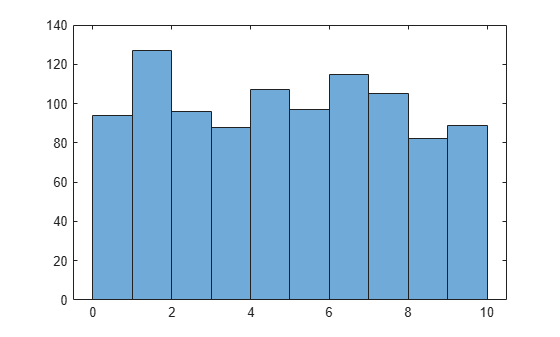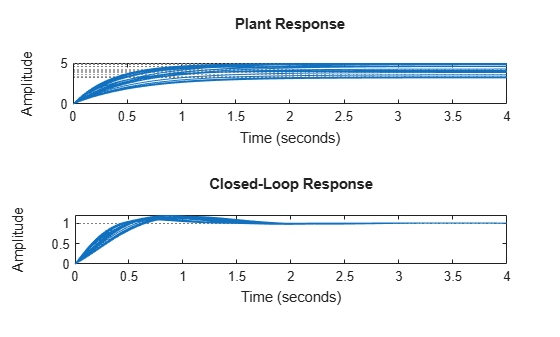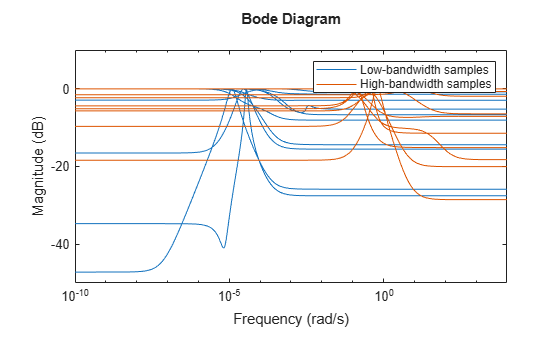Documentation

### This is machine translation

Mouseover text to see original. Click the button below to return to the English version of the page.

# uss/usample

Generate random samples of uncertain or generalized model

## Syntax

```B = usample(A);
B = usample(A,N)
[B,SampleValues] = usample(A,N)
[B,SampleValues] = usample(A,Names,N)
[B,SampleValues] = usample(A,Names1,N1,Names2,N2,...)
[B,SampleValues] = usample(A,N,Wmax)
[B,SampleValues] = usample(A,Names,N,Wmax)
```

## Description

`B = usample(A)` substitutes a random sample of the uncertain objects in `A`, returning a certain (i.e., not uncertain) array of size` [size(A)]`. The input `A` can be any uncertain element, matrix, or system, such as `ureal`, `umat`, `uss`, or `ufrd`. `A` can also be any generalized matrix or system, such as `genss` or `genmat`, that contains uncertain blocks and other types of Control Design Blocks (Control System Toolbox). If `A` contains non-uncertain control design blocks, these are unchanged in `B`. Thus, for example, `usample` applied to a `genss` with both tunable and uncertain blocks, the result is a `genss` array with only tunable blocks.

`B = usample(A,N)` substitutes `N` random samples of the uncertain objects in `A`, returning a certain (i.e., not uncertain) array of size` [size(A) N]`.

`[B,SampleValues] = usample(A,N)` additionally returns the specific sampled values (as a `Struct` whose field names are the names of `A'`s uncertain elements) of the uncertain elements. Hence, `B` is the same as `usubs(A,SampleValues)`.

`[B,SampleValues] = usample(A,Names,N)` samples only the uncertain elements listed in the `Names` variable (cell, or char array). If `Names` does not include all the uncertain objects in `A`, then `B` will be an uncertain object. Any entries of `Names` that are not elements of` A` are simply ignored. Note that `usample(A,fieldnames(A.Uncertainty),N)` is the same as `usample(A,N)`.

`[B,SampleValues] = usample(A,Names1,N1,Names2,N2,...)` takes `N1` samples of the uncertain elements listed in `Names1`, and `N2` samples of the uncertain elements listed in `Names2`, and so on. `size(B)` will equal ```[size(A) N1 N2 ...]```.

The scalar parameter `Wmax` in

```[B,SampleValues] = usample(A,N,Wmax) [B,SampleValues] = usample(A,Names,N,Wmax) [B,SampleValues] = usample(A,Names,N,Wmax) ```

affects how `ultidyn` elements within `A` are sampled, restricting the poles of the samples. If `A` is a continuous-time `uss` or `ufrd`, then the poles of sampled `GainBounded` `ultidyn` elements in `SampleValues` will each have magnitude <= `BW`. If `A` is a discrete-time, then sampled `GainBounded` `ultidyn` elements are obtained by Tustin transformation, using `BW/(2*TS)` as the (continuous) pole magnitude bound. In this case, `BW` should be `< 1`. If the `ultidyn` type is `PositiveReal`, then the samples are obtained by bilinearly transforming (see Normalizing Functions for Uncertain Elements) the `GainBounded` elements described above.

## Examples

collapse all

Create a real uncertain parameter, sample it, and plot a histogram of the sampled values.

```A = ureal('A',5); Asample = usample(A,500);```

Examine the size of the parameter and the sample array.

`size(A)`
```Uncertain real scalar. ```
`size(Asample)`
```ans = 1×3 1 1 500 ```

`A` is a scalar parameter. The dimensions of `Asample` reflect that `A` is a 1-by-1 parameter. Examine the data type of `Asample`.

`class(Asample)`
```ans = 'double' ```

The samples of the scalar parameter are numerical values.

Plot the histogram of sampled values.

`hist(Asample(:))`This example illustrates how to sample the open and closed-loop response of an uncertain plant model for Monte Carlo analysis.

Create two uncertain real parameters and an uncertain plant.

```gamma = ureal('gamma',4); tau = ureal('tau',.5,'Percentage',30); P = tf(gamma,[tau 1]);```

Create an integral controller based on the nominal values of plant uncertainties.

```KI = 1/(2*tau.Nominal*gamma.Nominal); C = tf(KI,[1 0]);```

Now create an uncertain closed-loop system.

`CLP = feedback(P*C,1);`

Sample the plant at 20 values, distributed uniformly about the `tau` and `gamma` parameter cube.

```[Psample1D,Values1D] = usample(P,20); size(Psample1D)```
```20x1 array of state-space models. Each model has 1 outputs, 1 inputs, and 1 states. ```

This sampling returns an array of 20 state-space models, each representing the closed-loop system within the uncertainty.

Now sample the plant at 10 values of `tau` and 15 values of `gamma`.

```[Psample2D,Values2D] = usample(P,'tau',10,'gamma',15); size(Psample2D)```
```10x15 array of state-space models. Each model has 1 outputs, 1 inputs, and 1 states. ```

Plot the step responses of the 1-D sampled plant.

```subplot(2,1,1); step(Psample1D)```Evaluate the uncertain closed-loop model at the same values using `usubs`, and plot the step response.

```subplot(2,1,2); step(usubs(CLP,Values1D))```To see the effect of limiting the bandwidth of sampled models with `Wmax`, create two `ultidyn` objects.

```A = ultidyn('A',[1 1]); B = ultidyn('B',[1 1]);```

Sample 10 instances of each, using a bandwidth limit of 1 rad/sec on `A`, and 20 rad/sec on `B`.

```Npts = 10; As = usample(A,Npts,1); Bs = usample(B,Npts,20);```

Plot 10-second step responses, for the two sample sets.

`step(As,'r',Bs,'b--',10)`The lower bandwidth limit on the samples of `A` results in generally slower step responses for those samples.